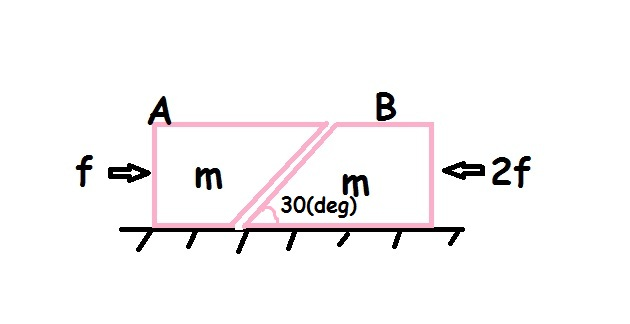# My first Mechanics Problems

Two blocks A and B each of mass are placed on a smooth horizontal surface. Two horizontal forces 'f' and '2f' are applied on the blocks A and B as shown in the figure. The block A does not slide on block B. then the normal force acting between two blocks is :

The answer is of the form 'k x f' where k is an integar . find 'k'.×